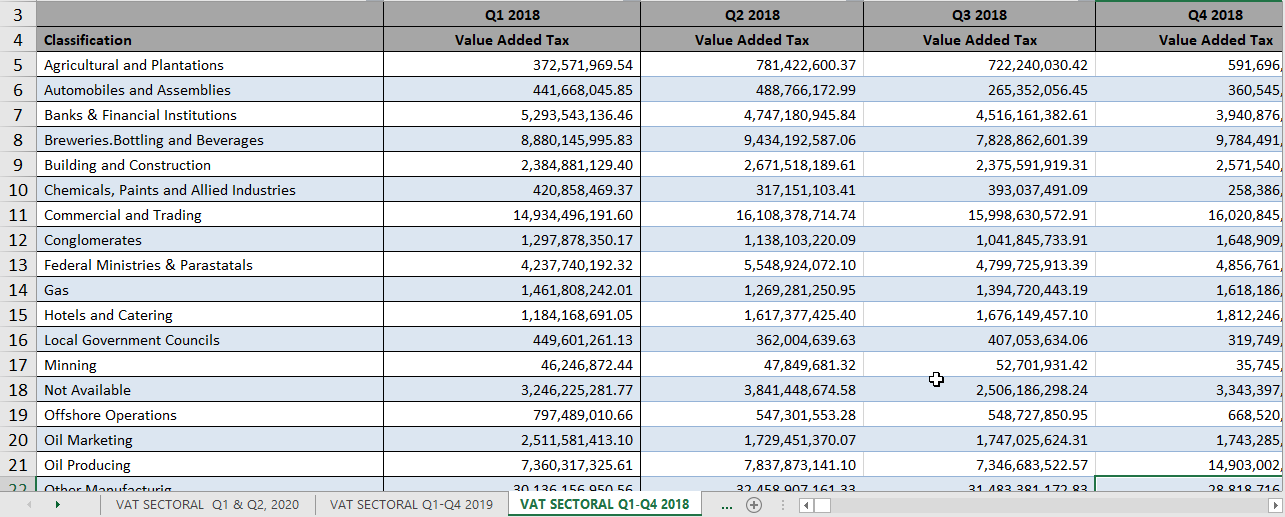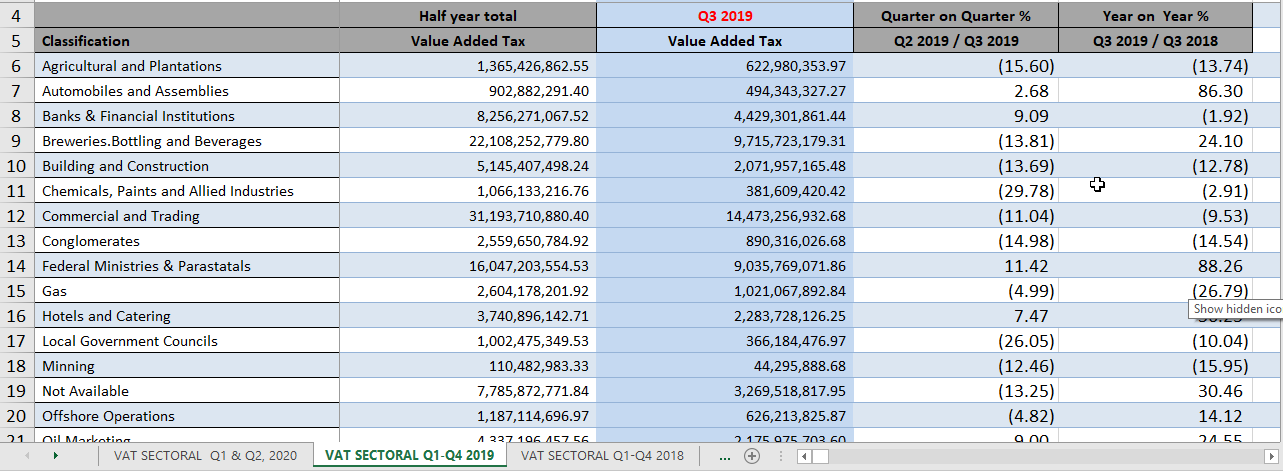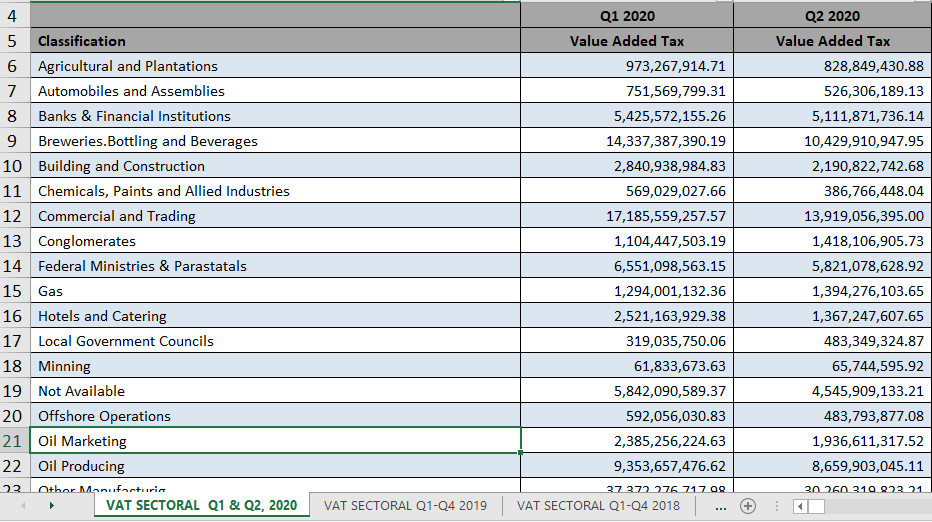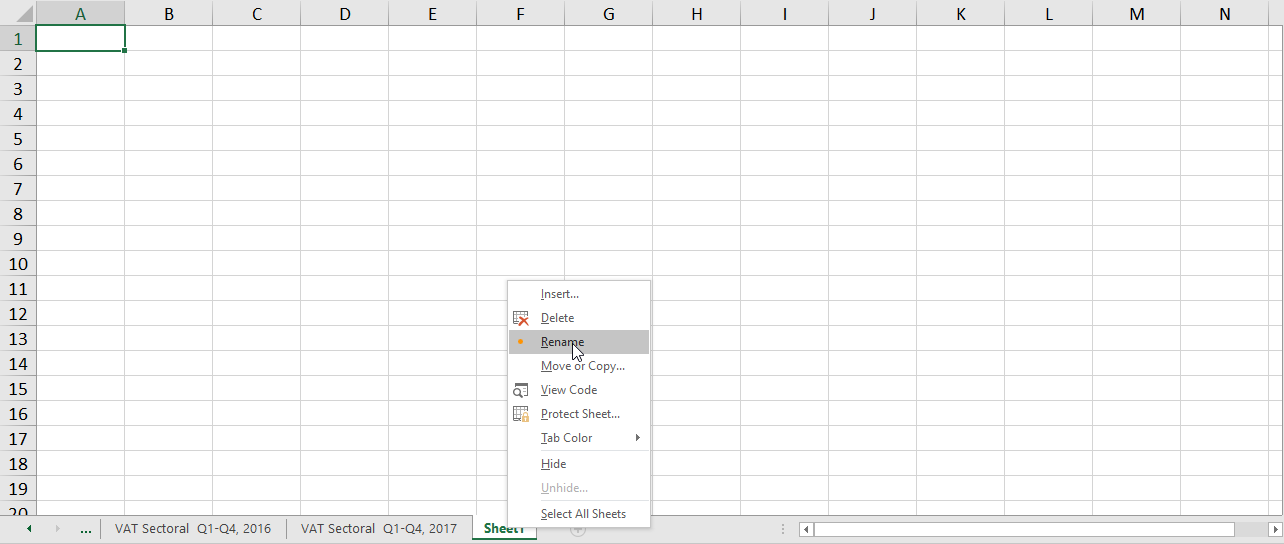VLOOKUP Function in ExcelFollowing the previous Excel article on Excel Wildcards, we will be discussing the usage of the commonly used Vlookup function.

Vlookup stands for ‘Vertical Lookup’. As the name Implies, Vlookup is a Microsoft Excel function that is usually used to lookup data organized vertically in a table. Vlookup supports approximate and exact matching, and wildcards (* ?) for partial matches. The Vlookup function always looks up a value in the leftmost column of a table and returns the corresponding value from a column to the right, and you look up a value by matching on the value from the column you want to match the values.

The Vlookup function searches for a certain value in a column (which is usually called a ‘table array)’, in order to return a value from a different column in the same row.  Vlookup function can also be used to merge different tables into one table in Excel.

Syntax

=VLOOKUP (value, table, col_index, [range_lookup])

value – This is the value to lookup in the first column of the table

table –  This simply refers to the table from which to retrieve value

col_index – This the number of the column in the table from which to retrieve a value

range_lookup – This can be either True or False match : [optional] TRUE = approximate match (default). FALSE = exact match.

In this article, we will be focusing on showing you how to merge different tables in Excel using the Vlookup function.

Note: In order to merge different tables/sheets together, there should be a variable/column that is common to all the tables. In the example used in this article, the Vlookup function will be applied to different tables in the workbook from 2013 to 2020 on the Nigeria Sectoral Distribution of Value Added Tax.

In these datasets, the common and constant variable among all of them is the first column which is the “Classification” column. See the images below to understand what a common variable is in this context.Judging from the above images there is a common constant variable across the years which is the Classification column. In this case, the lookup value will be the classification column.

Before applying the Vlookup function to the dataset you want to match the values on, make sure you give your tables a name for a more organised and easy application of the function.

Application of the Vlookup function

We will be working with the Nigeria Sectoral Distribution of Value Added Tax dataset. You can download the dataset by following the link below.

In the example below, we will use the Vlookup function to lookup the Distribution of Value Added Tax of the first quarter of each year and merge them into one table.

The first thing to do is to add a new sheet where we will merge the data we want to look up from the other sheets.

• Click on the plus (+) sign
• After adding the new sheet, right-click on the sheet and click on ‘Rename’ in the pop-up menu
• Give your new sheet a new name

Note: It is not compulsory to create a new sheet for this. You can add a new column in the existing sheet, however, to get a well-organised table, it is advisable to add a new sheet or a new Excel workbook.Next, you copy the values from the ‘Classification‘ column from any of the VAT datasheets into the new sheet created. The values in the classification column are almost constant across all the sheets. You get points for figuring out the slight difference in values.

Your new worksheet should look like this. Here, I named the new sheet “Merged_TableBelow is the output of the application of the Vlookup function using the constant variable (Classification column) to lookup 2017 Q1 data.

In the formula,

• A2 refers to the lookup value, taken from the Classification column.
• The lookup array is from the 2017 VAT worksheet. We have highlighted the range B3:G32 from the 2017 VAT worksheet.
• The column number is 2 because we want to retrieve the Q1 data from the 2017 worksheet.
• Finally, we choose a FALSE option to initiate an exact match.

Below is the final output after applying the Vlookup function to merge data for quarter 1 for the years 2017, 2018, and 2019.

Check this space next month for the Power BI Update for August 2020

We appreciate our readers and learners and would love to get your feedback.

Don’t forget to like, share the article and subscribe for more exciting content.

What next?

Data Analytics and Data Science are two of the top skills needed to navigate the 4th industrial revolution and are guaranteed to boost your career.

Join our next masterclass sessions to learn Data Analysis and Visualisation with Power BI.

To find out more about what we do, subscribe to our insights below or you can reach out to us directly.